# 06-240/Classnotes For Thursday October 5

### Scan of Tutorial notes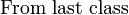$\mbox{From last class}{}_{}^{}$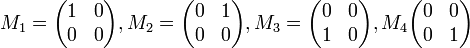$M_1=\begin{pmatrix}1&0\\0&0\end{pmatrix}, M_2=\begin{pmatrix}0&1\\0&0\end{pmatrix}, M_3=\begin{pmatrix}0&0\\1&0\end{pmatrix}, M_4\begin{pmatrix}0&0\\0&1\end{pmatrix}$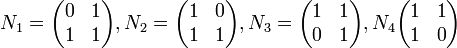$N_1=\begin{pmatrix}0&1\\1&1\end{pmatrix}, N_2=\begin{pmatrix}1&0\\1&1\end{pmatrix}, N_3=\begin{pmatrix}1&1\\0&1\end{pmatrix}, N_4\begin{pmatrix}1&1\\1&0\end{pmatrix}$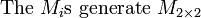$\mbox{The }M_i\mbox{s generate }M_{2\times 2}$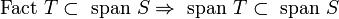$\mbox{Fact }T\subset\mbox{ span }S\Rightarrow \mbox{ span }T\subset\mbox{ span }S$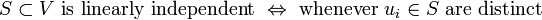$S\subset V\mbox{ is linearly independent }\Leftrightarrow \mbox{ whenever }u_i\in S\mbox{ are distinct}$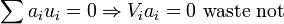$\sum a_iu_i=0\Rightarrow V_ia_i=0 \mbox{ waste not}$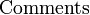$\mbox{Comments}{}_{}^{}$

1.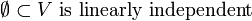$\emptyset\subset V\mbox{ is linearly independent}$
2.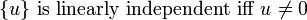$\lbrace u\rbrace\mbox{ is linearly independent iff }u_{}^{}\neq 0$
3.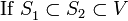$\mbox{If }S_1^{}\subset S_2\subset V$
1.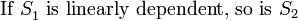$\mbox{If }S_1^{}\mbox{ is linearly dependent, so is }S_2$
2.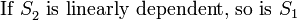$\mbox{If }S_2^{}\mbox{ is linearly dependent, so is }S_1$
3.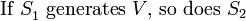$\mbox{If }S_1^{}\mbox{ generates }V\mbox{, so does }S_2$
4.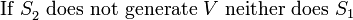$\mbox{If }S_2^{}\mbox{ does not generate }V\mbox{ neither does }S_1$
4.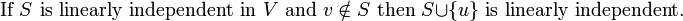$\mbox{If }S_{}^{}\mbox{ is linearly independent in }V\mbox{ and }v\notin S\mbox{ then }S\cup\lbrace u\rbrace\mbox{ is linearly independent.}$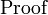$\mbox{Proof}{}_{}^{}$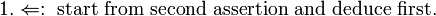$\mbox{1.}\Leftarrow:\mbox{ start from second assertion and deduce first.}$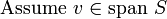$\mbox{Assume }v_{}^{}\in \mbox{span }S$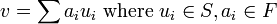$v=\sum a_iu_i\mbox{ where }u_i\in S, a_i\in F$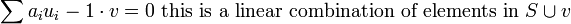$\sum a_iu_i-1\cdot v=0\mbox{ this is a linear combination of elements in }S\cup v$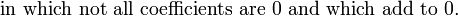$\mbox{ in which not all coefficients are }0 \mbox{ and which add to }0_{}^{}.$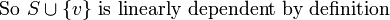$\mbox{So }S\cup \lbrace v\rbrace\mbox{ is linearly dependent by definition}$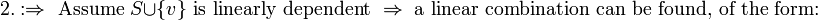$\mbox{2.}:\Rightarrow\mbox{ Assume }S\cup \lbrace v\rbrace\mbox{ is linearly dependent }\Rightarrow\mbox{ a linear combination can be found, of the form:}$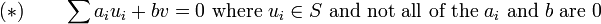$(*)\qquad\sum a_iu_i+bv=0\mbox{ where }u_i\in S\mbox{ and not all of the }a_i \mbox{ and }b \mbox{ are }0$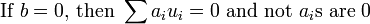$\mbox{If }b=0\mbox{, then }\sum a_iu_i=0\mbox{ and not }a_i\mbox{s are }0$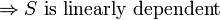${}_{}^{}\Rightarrow S \mbox{ is linearly dependent}$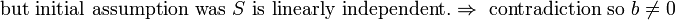${}_{}^{}\mbox{but initial assumption was }S\mbox{ is linearly independent.}\Rightarrow \mbox{ contradiction so }b\neq0$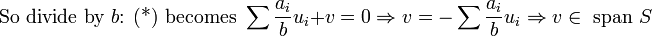$\mbox{So divide by }b\mbox{: (*) becomes }\sum\frac{a_i}{b}u_i + v = 0\Rightarrow v=-\sum\frac{a_i}{b}u_i\Rightarrow v\in \mbox{ span }S$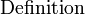$\mbox{Definition}{}_{}^{}$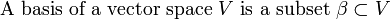${}_{}^{}\mbox{A basis of a vector space }V\mbox{ is a subset }\beta\subset V$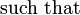${}_{}^{}\mbox{such that}$

1.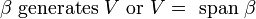${}_{}^{}\beta\mbox{ generates }V\mbox{ or }V=\mbox{ span }\beta$
2.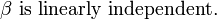${}_{}^{}\beta\mbox{ is linearly independent.}$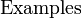$\mbox{Examples}{}_{}^{}$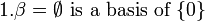$1. \beta=\emptyset{}_{}^{}\mbox{ is a basis of }\lbrace0\rbrace$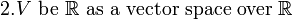$2. {}_{}^{}V\mbox{ be }\mathbb{R}\mbox{ as a vector space over }\mathbb{R}$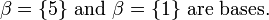$\qquad{}_{}^{}\beta=\lbrace5\rbrace\mbox{ and }\beta=\lbrace1\rbrace\mbox{ are bases.}$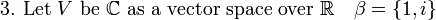$3.{}_{}^{}\mbox{ Let }V\mbox{ be }\mathbb{C}\mbox{ as a vector space over }\mathbb{R} \quad\beta=\lbrace1,i\rbrace$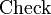$\qquad{}_{}^{}\mbox{Check}$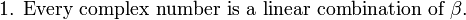$\qquad{}_{}^{}\mbox{1. Every complex number is a linear combination of }\beta.$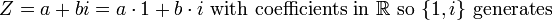$Z=a+bi=a\cdot 1+b\cdot i\mbox{ with coefficients in }\mathbb{R}\mbox{ so }\lbrace1,i\rbrace\mbox{ generates}$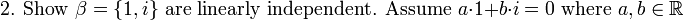$\qquad{}_{}^{}\mbox{2. Show }\beta=\lbrace1,i\rbrace\mbox{ are linearly independent. Assume }a\cdot 1+b\cdot i=0\mbox{ where }a,b\in\mathbb{R}$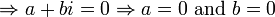${}_{}^{}\Rightarrow a+bi=0\Rightarrow a=0\mbox{ and } b=0$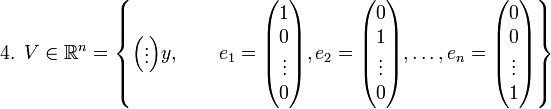${}_{}^{}\mbox{4. }V\in\mathbb{R}^n= \left\lbrace\begin{pmatrix}\vdots\end{pmatrix}y,\qquad e_1=\begin{pmatrix}1\\0\\\vdots\\0\end{pmatrix}, e_2=\begin{pmatrix}0\\1\\\vdots\\0\end{pmatrix},\ldots, e_n=\begin{pmatrix}0\\0\\\vdots\\1\end{pmatrix}\right\rbrace$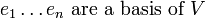${}_{}^{}e_1\ldots e_n\mbox{ are a basis of }V$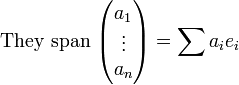${}_{}^{}\mbox{They span }\begin{pmatrix}a_1\\\vdots\\a_n\end{pmatrix}=\sum a_ie_i$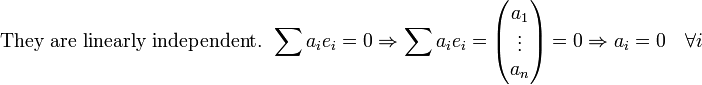${}_{}^{}\mbox{They are linearly independent. }\sum a_ie_i=0\Rightarrow \sum a_ie_i= \begin{pmatrix}a_1\\\vdots\\a_n\end{pmatrix}=0\Rightarrow a_i=0 \quad\forall i$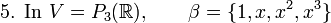${}_{}^{}\mbox{5. In }V=P_3(\mathbb{R}),\qquad \beta=\lbrace 1,x,x^2,x^3\rbrace$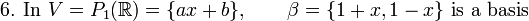${}_{}^{}\mbox{6. In }V=P_1(\mathbb{R})=\lbrace ax+b\rbrace,\qquad \beta=\lbrace 1+x,1-x\rbrace\mbox{ is a basis}$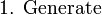${}_{}^{}\mbox{1. Generate }$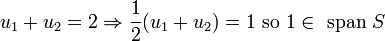$u_1+u_2=2\Rightarrow \frac{1}{2}(u_1+u_2)=1\mbox{ so }1 \in\mbox{ span }S$$u_1-u_2=2x\Rightarrow \frac{1}{2}(u_1-u_2)=x\mbox{ so }x \in\mbox{ span }S$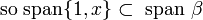${}_{}^{}\mbox{ so span}\lbrace 1,x\rbrace \subset\mbox{ span }\beta$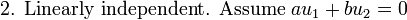${}_{}^{}\mbox{2. Linearly independent. Assume }au_1+bu_2=0$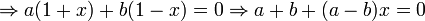$\Rightarrow a(1+x)+b(1-x)=0\Rightarrow a+b+(a-b)x=0$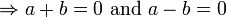${}_{}^{}\Rightarrow a+b=0\mbox{ and }a-b=0$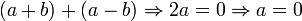$(a+b)+(a-b)\Rightarrow 2a=0\Rightarrow a=0$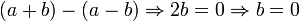$(a+b)-(a-b)\Rightarrow 2b=0\Rightarrow b=0$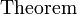$\mbox{Theorem}{}_{}^{}$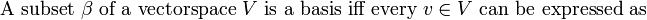${}_{}^{}\mbox{A subset }\beta\mbox{ of a vectorspace }V \mbox{ is a basis iff every }v\in V\mbox{ can be expressed as}$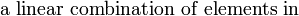${}_{}^{}\mbox{a linear combination of elements in }$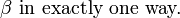${}_{}^{}\beta \mbox{ in exactly one way.}$$\mbox{Proof}{}_{}^{}$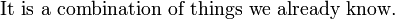${}_{}^{}\mbox{It is a combination of things we already know.}$

1.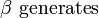${}_{}^{}\beta\mbox{ generates}$
2.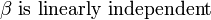${}_{}^{}\beta\mbox{ is linearly independent}$